Ion counters measure the concentration of small ion charge carriers in the air (as we will see they can only measure one polarity of charge carrier at a time).  A conductivity meter measures the air's conductivity.  And, actually, it isn't able to measure the total conductivity because both positively and negatively charged small ions make the air conductive.  A conductivity meter is only able to measure the contribution that small ions of a single polarity make.

Ions counters and conductivity meters often make use of the cylindrical capacitor geometry shown below.  The outer electrode is connected to plus or minus voltage V, the inner electrode is grounded.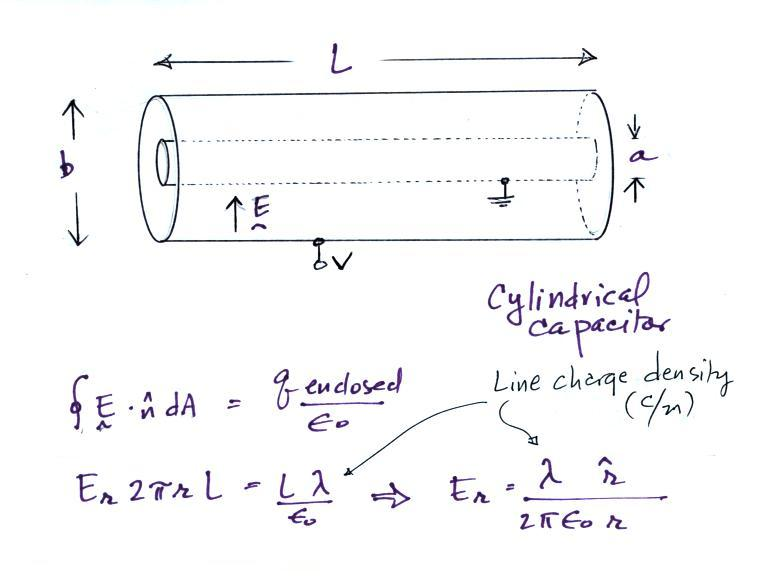If we assume that we are looking a just a small section in the middle of a much longer cylinder, the E field will have just a radial component, Er.  The solution above, obtained using Gauss' Law, is the same as the field around an infinitely long line of charge with uniform line charge density.

Next we will try to find an expression for the electric field in terms of the potential difference between the two cylinders.If the outer cylinder is connected to positive potential, the grounded inner cylinder will have negative charged induced on it.  The E field will point inward as shown in the figure above.

It is a relatively easy matter to determine the capacitanceLet's look first at how an ion counter works.  An ion counter will measure the concentration of small ions in the air.The electric field will cause a  positively charged small ion entering the cylindrical capacitor at the left to drift toward the inner conductor with a drift velocity (vd) In time dt, the small ion will drift dr (Point 1).  The drift velocity, Point 2, is just the electrical mobility, Be, times the electric field.  T at Point 3 is the time it will take the small ion to drift from the outer cylinder to the inner cylinder.Now in order to be "counted" the small ion must make it to the center conductor before it travels a distance L, the length of the cylindrical capacitor.This means (Point 4) that T must be less than L/u, where u is the speed at which the air is traveling along the length of the cylindrical capacitor (we assume u is uniform, that there is no dependence on r).

Mobile ions are more likely to make it to the center electrode.  So another way of looking at this is in terms of a critical mobility, Bc.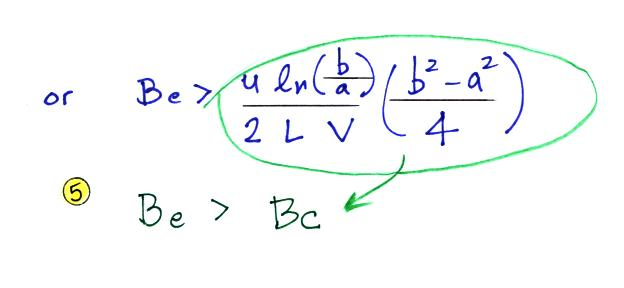Ions with a mobility Be greater than Bc will be collected, the others won't.  The volumetric flow rate is an easier parameter to measure than the horizontal speed.  So we can rewrite Bc in terms of flow rate.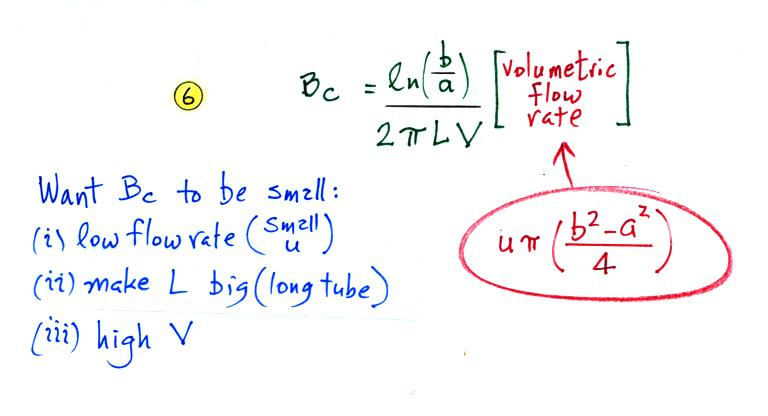We want Bc to be small so that all of the small ions have a mobility greater than Bc and can be counted.  Clearly the lower the flow rate and the longer the tube, the more time the small ions will spend in the capacitor and the more likely they will be collected.  Increasing the potential difference between the two cylinders will increase the strength of the electric field and the inward drift velocity of the small ions.We'll assume that all of the small ions of one polarity are collected by the center electrode as they pass through the cylinder.  The current flowing to the center electrode would then be the product of the small ion concentration, the charge per small ions, and the volumetric flow rate.  Later in this lecture we will look at instrumentation that could be used to measure this (small) current.

When functioning as a conducitivty meter, only the small ions in a portion of the volume of air flowing through the cylindrical capacitor are collected (the green shaded volume in the figure below).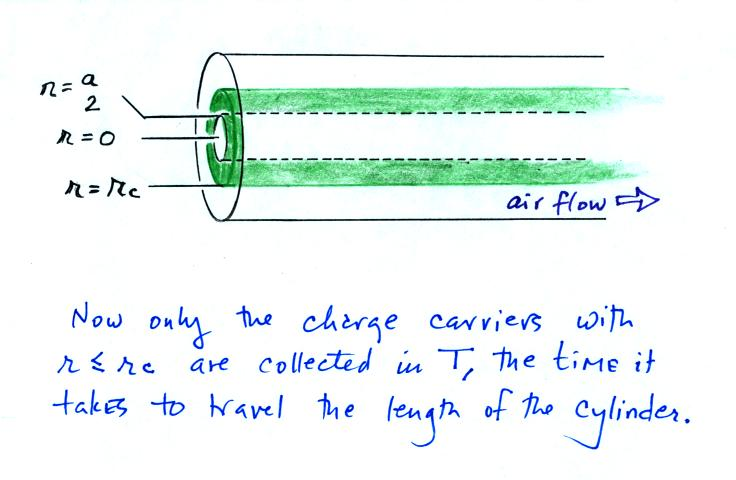On an earlier figure (Point 3) we determined the time, T, needed to travel from the outer electrode to the inner electrode (from b/2 to a/2).  We'll write down the expression again but substitute rc for b/2 (see Point 7 below).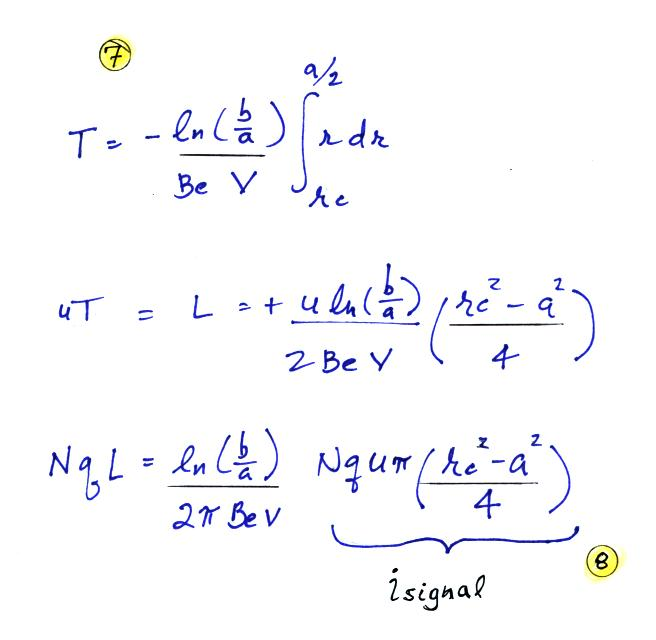The last term at Point 8 is the rate at which the green shaded volume is flowing through the cylinder times N q.  This is the charge collected at the inner conductor per unit time and is the signal current.  Let's solve the expression for isignal and then try to relate the signal current to conductivity.We substitute in for capacitance and next we recall that N Be q is conductivity.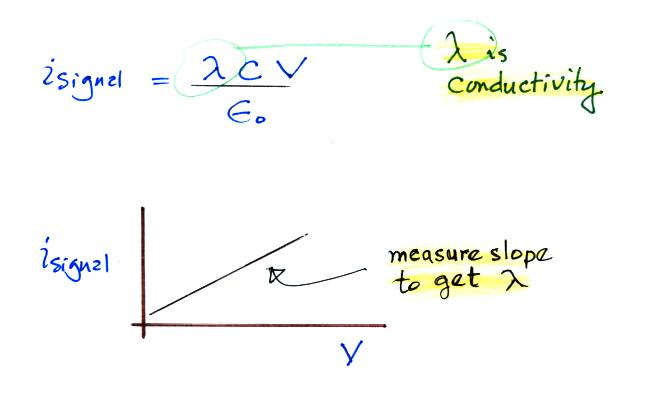There is a linear relationship between isignal and V.  The slope of a plot of isignal vs V should provide an estimate of conductivity.
And again because we collect only one polarity of small ion we aren't measuring the total conductivity.  The total conductivity depends on charge carriers of both polarities.

The following figure shows a conventional ion counter/conductivity meter design and an op-amp circuit that could be used to measure the signal current (Vout  = signal current x R).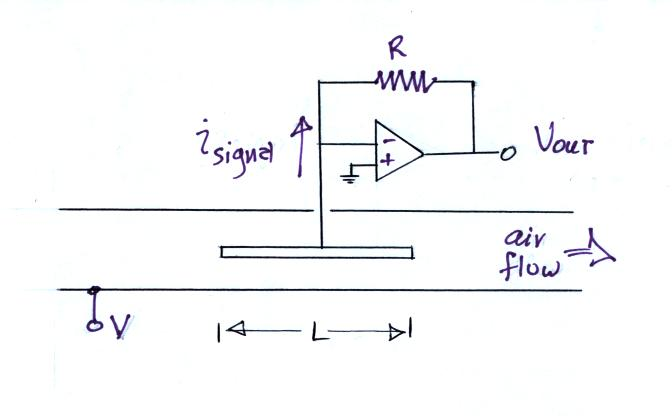Note how the op-amp keeps the center conductor at ground potential.  Because the signal current is very small, a large feedback resistor is needed in the op-amp circuit (1013 ohms was used in an "instrument" demonstrated in class).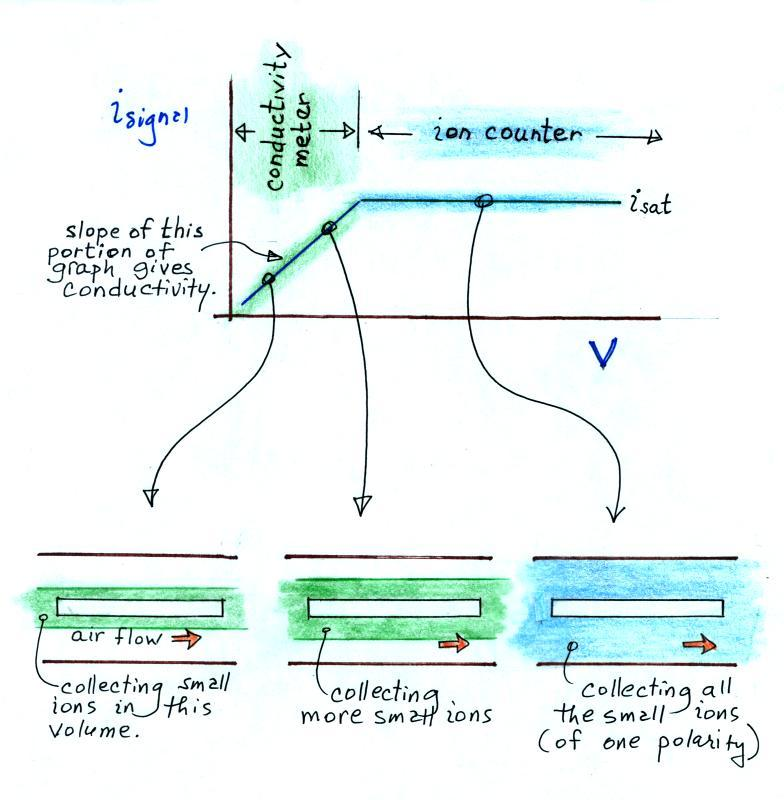In this last figure we get a better idea of how this instrument can function as either a conductivity meter or an ion counter and how it transitions from one to the other.

For a given rate of air flow through the cylindrical capacitor we monitor the signal current as the potential difference between the outer and inner conductors is increased.  As V increases small ions in a growing volume of air are collected and measured.  The signal current increases.  Eventually all of the small ions are collected and the signal current flattens out (saturates).

The slope of the linearly increasing, early portion of the plot (shaded green above) provides an estimate of conductivity.  The amplitude of the signal current, once it has flattened out (blue) can be related to small ion concentration.

A common household ionization-type smoke detectors is really just a very basic conductivity meter.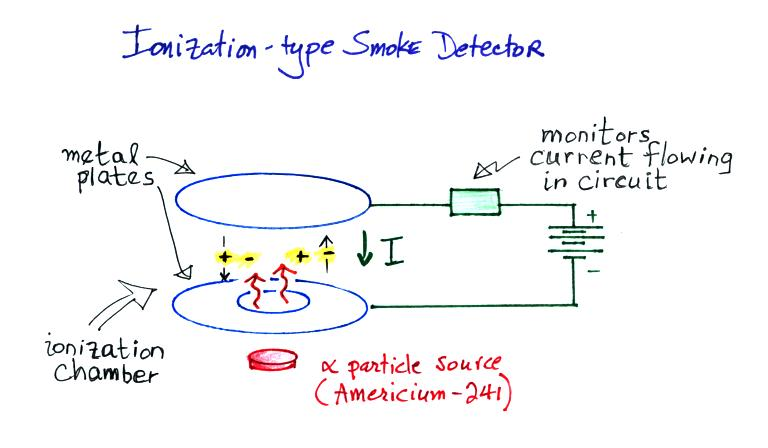Alpha particles from a small amount of radioactive Americium-241 ionizes the air between two metal plates.  The plates are connected to a battery and the voltage difference causes a weak current to flow between the plates.

The current flowing through the ionization chamber drops significantly when smoke enters the chamber.  This is because the charge carriers quickly stick to any smoke particles that enter the ionization chamber and suffer a large drop in their electrical mobility.  The drop in current is sensed and used to sound the alarm.